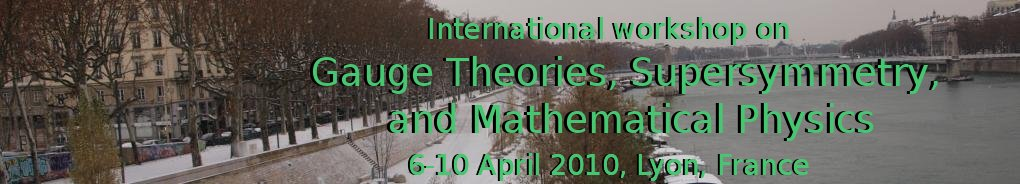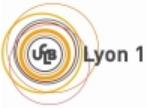NEW:
Materials are available on-line.
International workshop on
Gauge Theories, Supersymmetry, and Mathematical Physics.
6-10 April 2010, Lyon, France

# Materials

## Plenary lectures

### G. Cardoso BPS black holes, the Hesse potential, and the topological string

The free energy of four-dimensional BPS black holes is given by the generalized Hesse potential. We construct new variables for the Hesse potential, and we exhibit the relation of this Hesse potential with the functions computed on the topological string side.
See the slides### B. de Wit On BPS black hole entropy

See the slides### D. Roytenberg The modular class of a differential graded manifold

The modular class is the simplest intrinsic characteristic class that can be defined for any Q-manifold. For differential graded manifolds, it has a nice interpretation in terms of the tangent complex; this generalizes the construction, due to Evens, Lu and Weinstein, of the modular class of a Lie algebroid, to higher Lie algebroids. For Courant algebroids with a non-degenerate pseudometric, the modular class vanishes.
See the notes### V. Ovsienko Quaternions, octonions and beyond: a superalgebra point of view

See the article### P. Sundell Quantization, geometry and background (in)dependence in high-spin theory

We describe an action principle for the Type A and B Vasiliev systems. It is based on a sigma model for a "maximal" set of auxiliary fields and dual potentials. The Lagrangian consists of an unbroken bulk piece plus soft-symmetry breaking "impurities" that drive the theory into various broken phases. In particular, we define high-spin generalized Riemannian geometries characterized by observables depending explicitly on a high-spin vielbein, such as minimal areas and homotopy charges, and propose a corresponding impurity given by a full counterpart of the Fradkin-Vasiliev action. We finally comment on how the UV softness of the theory fits naturally into the conjectured AdS/CFT correspondence and discuss how effective four-dimensional low-spin theories (including gravity) can arise on "Higgs branches" of high-spin theories.
See the slides### A. Tomasiello The geometry of Romans mass

The "Romans mass" is a discrete parameter in type IIA supergravity. It is perhaps the most mysterious piece of the theory: for example, it is not known whether it has an M-theoretic interpretation. I will review some recent progress on the effect it has on the geometry of the internal space for supersymmetric compactifications, and on its holographic interpretation in terms of a Chern-Simons theory.
See the slides### U. Theis D-brane instantons from string dualities

See the slides### T. Voronov On a non-Abelian Poincare lemma and Lie agebroids

I shall discuss a generalization of the well-known statement that a Lie algebra valued 1-form satisfying the Maurer-Cartan equation is a "pure gauge". If one considers arbitrary odd (pseudo)differential forms with values in a Lie superalgebra, there is a non-Abelian version of the homotopy identity. In particular, an odd form satisfying the Maurer-Cartan equation on a contractible domain is gauge-equivalent to a constant. This can be applied to Lie algebroids and their non-linear analogs.
See the slides

## Short talks

### N. Boulanger Cubic interactions of higher-spin gauge fields

We review some recent and less recent progresses on the understanding of interacting higher-spin theory, mostly at the action level and for cubic vertices.
See the slides### P. Karndumri AdS_3 vacua and RG flows in three dimensional gauged supergravity

We study some vacua of N=4 (SO(4)xT^6)^2 gauged supergravity in three dimensions. The target space for the scalar fields is the product of two quaternionic manifolds, (SO(4,4)/SO(4)xSO(4)). Many supersymmetric AdS_3 vacua have been found, and, in particular, we have found RG flow solutions connecting some of these vacua namely one between (3,1) vacua and one between (2,0) vacua. The flows respect the c-theorem for the RG flows in 2D field theories.
See the slides
See the article### S. Slizovskiy New observables in instantonic topological field theories

We consider topological quantum mechanics, which is characterized by exact localization on instanton trajectories. The new observables correspond to arbitrary jumps of trajectories along fibers of a fibration or along a cycle in the group of diffeomorphisms. The examples and applications include correlators that compute linking numbers of fibers; and averaging over the loop rotations for QM on the loop space. (based on joint work with Andrei Losev 0911.2928)
See the slides

R. Kashaev The volume conjecture and analytic continuation of quantum Teichmuller theory The talk will be a survey of the volume conjecture and its connection with quantum Chern-Simons theory through the analytically continued quantum Teichmuller theory.
A. Kotov DG Lie groups, bundles, and characteristic classes I will discuss the notion of a graded differential Lie (super)group, that is, a graded super Lie group supplied with a compatible homological vector field, and its relation to gauge theory.
C. Laurent-Gengoux Gerbes, and connections : the Lie groupoid point of view I shall make a review of a joined work with M. Stienon and P. Xu viewing connections on gerbes with the help of Lie groupoids (and Lie groupoid extensions) and explain why it is stricly equivalent to the point of view developped by L. Breen and B. Messing.
P. Mnev One-dimensional simplicial Chern-Simons theory We will discuss one-dimensional toy version of Chern-Simons theory. We will construct its simplicial version that comprises both the features of low-energy effective gauge theory and of a topological quantum field theory in the sense of Atiyah. This talk is based on joint work with A.Alekseev.
T. Mohaupt From Harmonic Maps to Black Hole Solutions The problem of constructing static solutions in theories of gravity and matter can often be reduced to the problem of constructing a harmonic map from space-time into an auxiliary pseudo-Riemannian manifold. Explicit solutions similar to those known in supergravity can be obtained if the metric of this auxiliary space has a potential and satisfies certain scaling properties. We will discuss two applications: the construction of multi-centered extremal black hole solutions and the deformation of (single-centered) extremal solutions into non-extremal solutions.
P. Sundell Quantization, geometry and background (in)dependence in high-spin theory We describe an action principle for the Type A and B Vasiliev systems. It is based on a sigma model for a "maximal" set of auxiliary fields and dual potentials. The Lagrangian consists of an unbroken bulk piece plus soft-symmetry breaking "impurities" that drive the theory into various broken phases. In particular, we define high-spin generalized Riemannian geometries characterized by observables depending explicitly on a high-spin vielbein, such as minimal areas and homotopy charges, and propose a corresponding impurity given by a full counterpart of the Fradkin-Vasiliev action. We finally comment on how the UV softness of the theory fits naturally into the conjectured AdS/CFT correspondence and discuss how effective four-dimensional low-spin theories (including gravity) can arise on "Higgs branches" of high-spin theories.
M. Volkov Superconducting non-Abelian vortices in Weinberg--Salam theory -- electroweak thunderbolts We present a detailed analysis of classical solutions in the bosonic sector of the electroweak theory which describe vortices carrying a constant electric current I. These vortices exist for any value of the Higgs boson mass and for any weak mixing angle, and in the zero current limit they reduce to Z strings. Their current is produced by the condensate of vector W bosons and typically it can attain billions of amperes. It seems that the current can be arbitrarily large, due to the scale invariance of the vector boson condensate. Finite vortex segments can transfer electric charge between different regions of space, similarly to thunderbolts. It is also possible that they can form loops stabilized by the centrifugal force -- electroweak vortons.
T. Voronov On a non-Abelian Poincare lemma and Lie agebroids I shall discuss a generalization of the well-known statement that a Lie algebra valued 1-form satisfying the Maurer-Cartan equation is a ?pure gauge? If one considers arbitrary odd (pseudo)differential forms with values in a Lie superalgebra, there is a non-Abelian version of the homotopy identity. In particular, an odd form satisfying the Maurer-Cartan equation on a contractible domain is gauge-equivalent to a constant. This can be applied to Lie algebroids and their non-linear analogs.
Short talks A. Mikovic Gauge-invariant actions for 2-BF theories We review 2-groups and their relevance for physics. Then we explain how to construct a gauge invariant action for a theory of 2-form and 1-form non-abelian gauge fields which comes from a BF theory based on a 2-group.Contact the webmaster